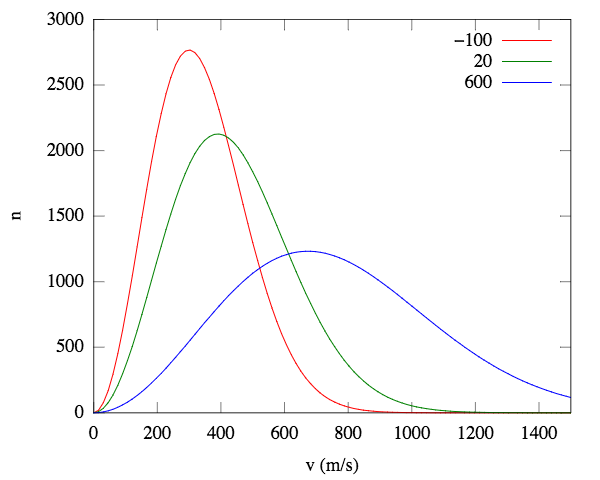## Distribution of Molecular Speeds and Collision Frequency

#### Learning Objective

• Identify the relationship between velocity distributions and temperature and molecular weight of a gas.

#### Key Points

• Gaseous particles move at random speeds and in random directions.
• The Maxwell-Boltzmann Distribution describes the average speeds of a collection gaseous particles at a given temperature.
• Temperature and molecular weight can affect the shape of Boltzmann Distributions.
• Average velocities of gases are often expressed as root-mean-square averages.

#### Terms

• quantathe smallest possible packet of energy that can be transferred or absorbed
• velocitya vector quantity that denotes the rate of change of position with respect to time or a speed with a directional component

According to the Kinetic Molecular Theory, all gaseous particles are in constant random motion at temperatures above absolute zero. The movement of gaseous particles is characterized by straight-line trajectories interrupted by collisions with other particles or with a physical boundary. Depending on the nature of the particles’ relative kinetic energies, a collision causes a transfer of kinetic energy as well as a change in direction.

## Root-Mean-Square Velocities of Gaseous Particles

Measuring the velocities of particles at a given time results in a large distribution of values; some particles may move very slowly, others very quickly, and because they are constantly moving in different directions, the velocity could equal zero. (Velocity is a vector quantity, equal to the speed and direction of a particle) To properly assess the average velocity, average the squares of the velocities and take the square root of that value. This is known as the root-mean-square (RMS) velocity, and it is represented as follows:

$\bar{v}=v_{rms}=\sqrt{\frac{3RT}{M_m}}$

$KE=\frac{1}{2}mv^2$

$KE=\frac{1}{2}mv^2$

In the above formula, R is the gas constant, T is absolute temperature, and Mm is the molar mass of the gas particles in kg/mol.

## Energy Distribution and Probability

Consider a closed system of gaseous particles with a fixed amount of energy. With no external forces (e.g. a change in temperature) acting on the system, the total energy remains unchanged. In theory, this energy can be distributed among the gaseous particles in many ways, and the distribution constantly changes as the particles collide with each other and with their boundaries. Given the constant changes, it is difficult to gauge the particles’ velocities at any given time. By understanding the nature of the particle movement, however, we can predict the probability that a particle will have a certain velocity at a given temperature.

Kinetic energy can be distributed only in discrete amounts known as quanta, so we can assume that any one time, each gaseous particle has a certain amount of quanta of kinetic energy. These quanta can be distributed among the three directions of motions in various ways, resulting in a velocity state for the molecule; therefore, the more kinetic energy, or quanta, a particle has, the more velocity states it has as well.

If we assume that all velocity states are equally probable, higher velocity states are favorable because there are greater in quantity. Although higher velocity states are favored statistically, however, lower energy states are more likely to be occupied because of the limited kinetic energy available to a particle; a collision may result in a particle with greater kinetic energy, so it must also result in a particle with less kinetic energy than before.

## Maxwell-Boltzmann Distributions

Using the above logic, we can hypothesize the velocity distribution for a given group of particles by plotting the number of molecules whose velocities fall within a series of narrow ranges. This results in an asymmetric curve, known as the Maxwell-Boltzmann distribution. The peak of the curve represents the most probable velocity among a collection of gas particles.

Velocity distributions are dependent on the temperature and mass of the particles. As the temperature increases, the particles acquire more kinetic energy. When we plot this, we see that an increase in temperature causes the Boltzmann plot to spread out, with the relative maximum shifting to the right.Effect of temperature on root-mean-square speed distributionsAs the temperature increases, so does the average kinetic energy (v), resulting in a wider distribution of possible velocities. n = the fraction of molecules.

Larger molecular weights narrow the velocity distribution because all particles have the same kinetic energy at the same temperature. Therefore, by the equation $KE=\frac{1}{2}mv^2$, the fraction of particles with higher velocities will increase as the molecular weight decreases.